# Tag: machine learning

## k nearest neighbors

Computers can automatically classify data using the k-nearest-neighbor algorithm.

For instance: given the sepal length and width, a computer program can determine if the flower is an Iris Setosa, Iris Versicolour or another type of flower.

Related courses

Dataset

Each plant has unique features: sepal length, sepal width, petal length and petal width. The measurements of different plans can be taken and saved into a spreadsheet.

The type of plant (species) is also saved, which is either of these classes:

• Iris Setosa (0)

• Iris Versicolour (1)

• Iris Virginica (2)

Put it all together, and we have a dataset: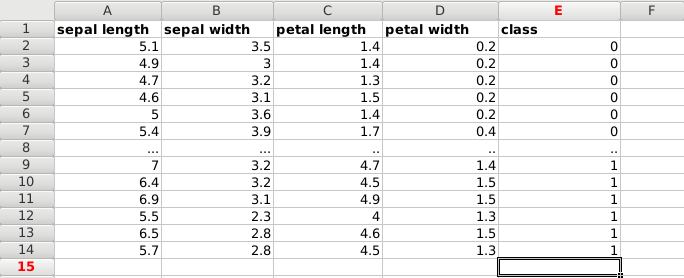We load the data. This is a famous dataset, it’s included in the module. Otherwise you can load a dataset using python pandas.

X contains the first two features, being the rows sepal length and sepal width. The Y list contains the classes for the features.

Plot data
We will use the two features of X to create a plot. Where we use X[:,0] on one axis and X[:,1] on the other.

This will output the data: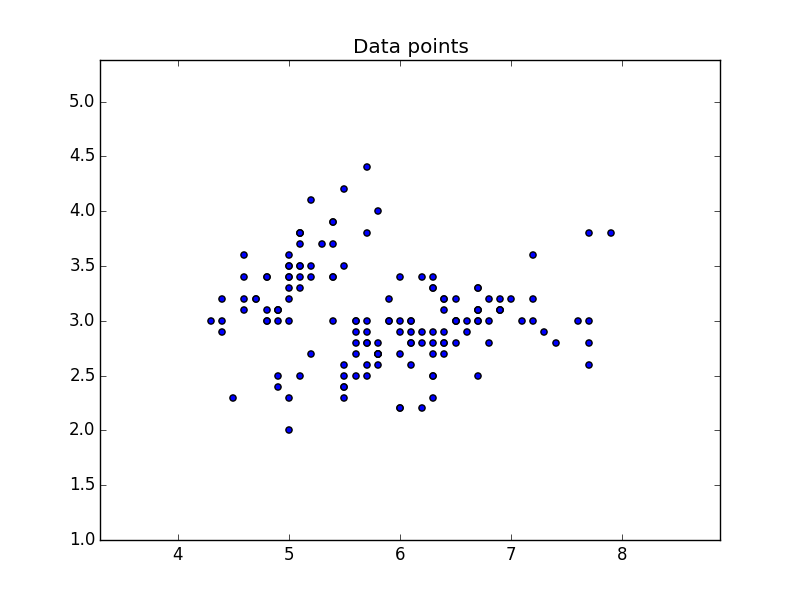Classify with k-nearest-neighbor
We can classify the data using the kNN algorithm. We create and fit the data using:

And predict the class using

This gives us the following code:

which outputs the plot using the 3 classes: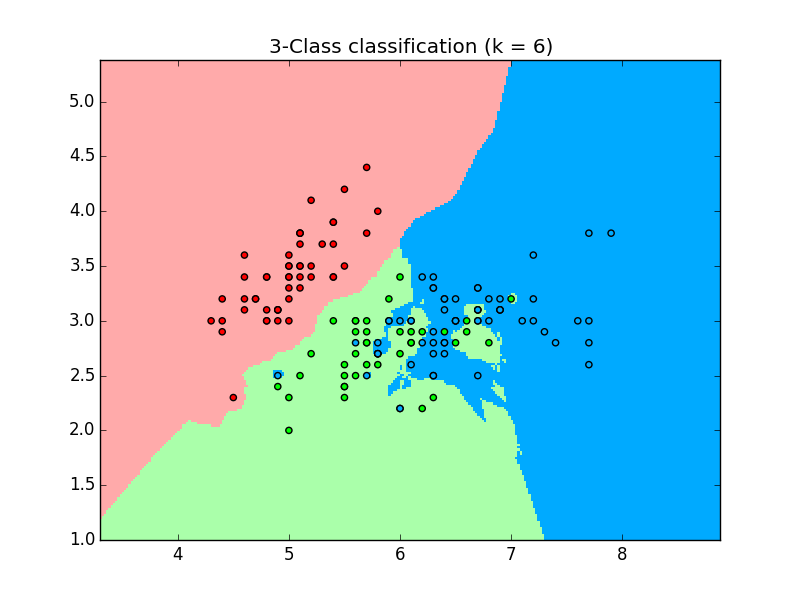Prediction
We can use this data to make predictions. Given the position on the plot (which is determined by the features), it’s assigned a class. We can put a new data on the plot and predict which class it belongs to.

The code below will make prediction based on the input given by the user:

## Linear Regression

How does regression relate to machine learning?

Given data, we can try to find the best fit line. After we discover the best fit line, we can use it to make predictions.

Data can be any data saved from Excel into a csv format, we will use Python Pandas to load the data.

Related courses

Required modules
You shoud have a few modules installed:

You can choose the graphical toolkit, this line is optional:

We start by loading the modules, and the dataset. Without data we can’t make good predictions.

The first step is to load the dataset. The data will be loaded using Python Pandas, a data analysis module. It will be loaded into a structure known as a Panda Data Frame, which allows for each manipulation of the rows and columns.

We create two arrays: X (size) and Y (price). Intuitively we’d expect to find some correlation between price and size.

The data will be split into a trainining and test set. Once we have the test data, we can find a best fit line and make predictions.

Finally we plot the test data.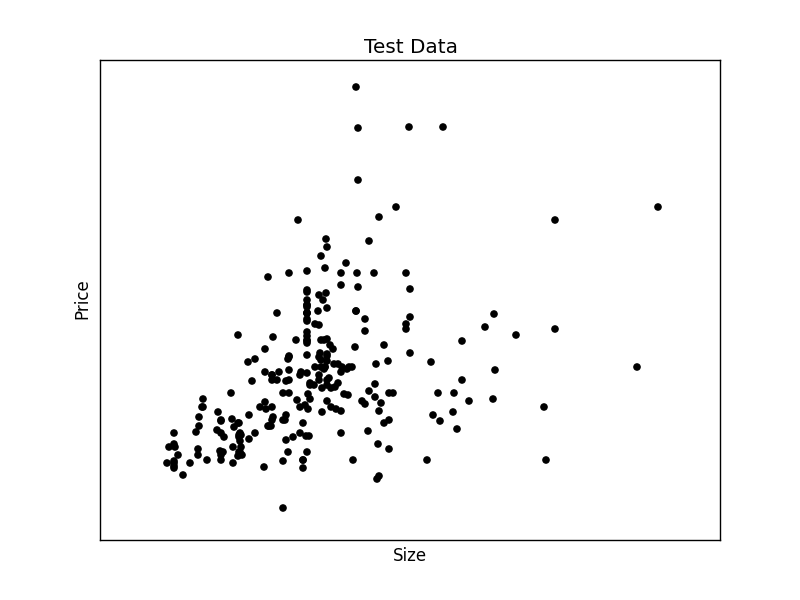We have created the two datasets and have the test data on the screen. We can continue to create the best fit line:

This will output the best fit line for the given test data.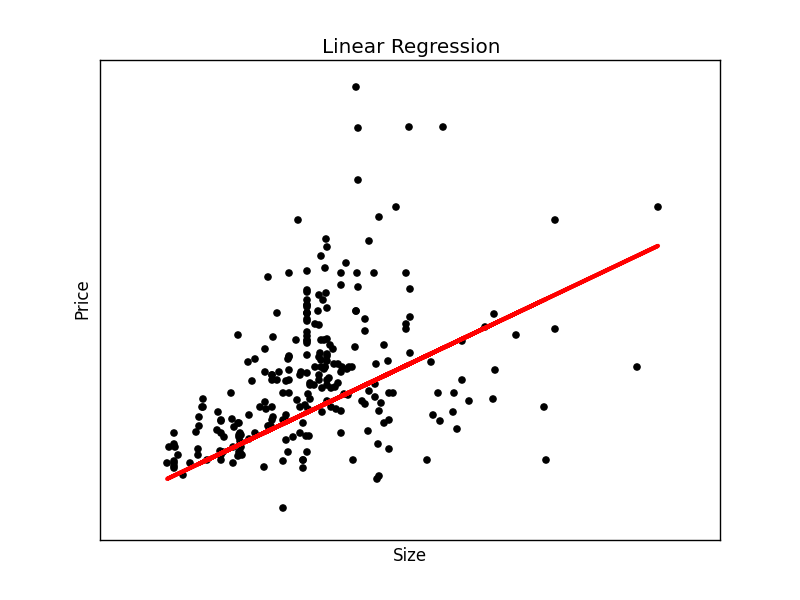To make an individual prediction using the linear regression model: